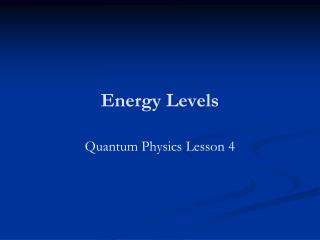DownloadDownload PresentationEnergy Levels

# Energy Levels

Télécharger la présentation## Energy Levels

- - - - - - - - - - - - - - - - - - - - - - - - - - - E N D - - - - - - - - - - - - - - - - - - - - - - - - - - -
##### Presentation Transcript

1. Energy Levels Quantum Physics Lesson 4

2. Learning Objectives • Explain what is meant by the term energy level. • Describe what happens when excited atoms de-excite. • Calculate the energy of emitted photons using the equation hf = E1 - E2. • Explain how a fluorescent tube works  HWK

3. Homework • Use books and the internet to research how fluorescent tubes work. • How do fluorescent tubes work? • Why are they used? • Use the words electrons, atoms, photons, ionisation and excitation in your answer. • Do not copy and paste  put in your own words! • Reference your work properly.

4. What is Quantisation? • Calculate the gravitational potential energy of your sweet to win it. • Notice that each sweet can only have a certain specific energy determined by the flat surfaces in the room. • When something can only take certain discrete values we say it is quantised e.g. shoe size.

5. Definitions Ground State Excited State An energy input raises the electrons to higher energy levels. The lowest energy level of an atom. When one or more of an atom’s electrons moves to an outer shell at higher energy.

6. Energy Levels • The electrons in an atom are trapped because they are attracted to the protons in the nucleus. • Their energies are determined by which shell they are in: nearer the nucleus = less energy. • But just like the sweets resting on the desk, each electron can only take certain discrete energies which correspond to the shells or orbits.

7. Video BBC The Atom – Jim Al-Khalili 3/6 0:00-9:10

8. Quantisation A quantum mechanical system or particle that is bound, confined spatially, can only take on certain discrete values of energy, as opposed to classical particles, which can have any energy. These values are called energy levels. The term is most commonly used for the energy levels of electrons in atoms or molecules, which are bound by the electric field of the nucleus. The energy spectrum of a system with energy levels is said to be quantised.

9. Energy Levels • “Why do the states have negative energy?” • This is because the zero of energy is considered to be that of a free electron 'just outside' the atom. • All energy states 'below' this – i.e. within the atom are therefore negative. • Energy must be put into the atom to raise the electron to the 'surface' of the atom and allow it to escape.

10. Well Analogy • Imagine you are stuck down a hole…with a number of ledges. • You could try to jump to get out but unless you jump high enough to reach one of the ledges you will just fall back down  releasing energy as sound energy. • It’s a bit like this in the atom the electrons need the ionisation energy to get out.

11. De-excitation • Whenever these is a vacancy in a shell an electron from a outer shell moves down to fill it. • When this happens the electron emits a photon. • The photon has an energy equal to the difference between the energy levels.

12. De-excitation

13. Equation In words:- In Symbols:- Note that E1 and E2 refer to the energies of the energy levels and hf is the energy of the photon emitted when an electron falls from the higher level to the lower level.

14. Excitation using Photons • An electron in an atom can absorb a photon and move to a higher energy level  But only if the photon energy matches the difference between the energy levels. This is not true for ionisation; as long as the energy of the incoming photon is greater than the ionisation energy, the electron can escape.

15. Electron in orbit round a nucleus in an atom Energy input. The electron is excited and rises to a higher energy level (shell). The electron falls back to its original energy level and energy is emitted in the form of radiation. The bigger the drop the greater the energy emitted and the shorter wavelength the radiation has (blue light).

16. Fluorescence • When an electron in a higher energy level de-excites it can either:- • Go straight down to the ground state  one photon. • Cascade down several energy levels  several photons, each of lower energy. • An atom can absorb UV but emit visible light.

17. The energy levels and spectra series